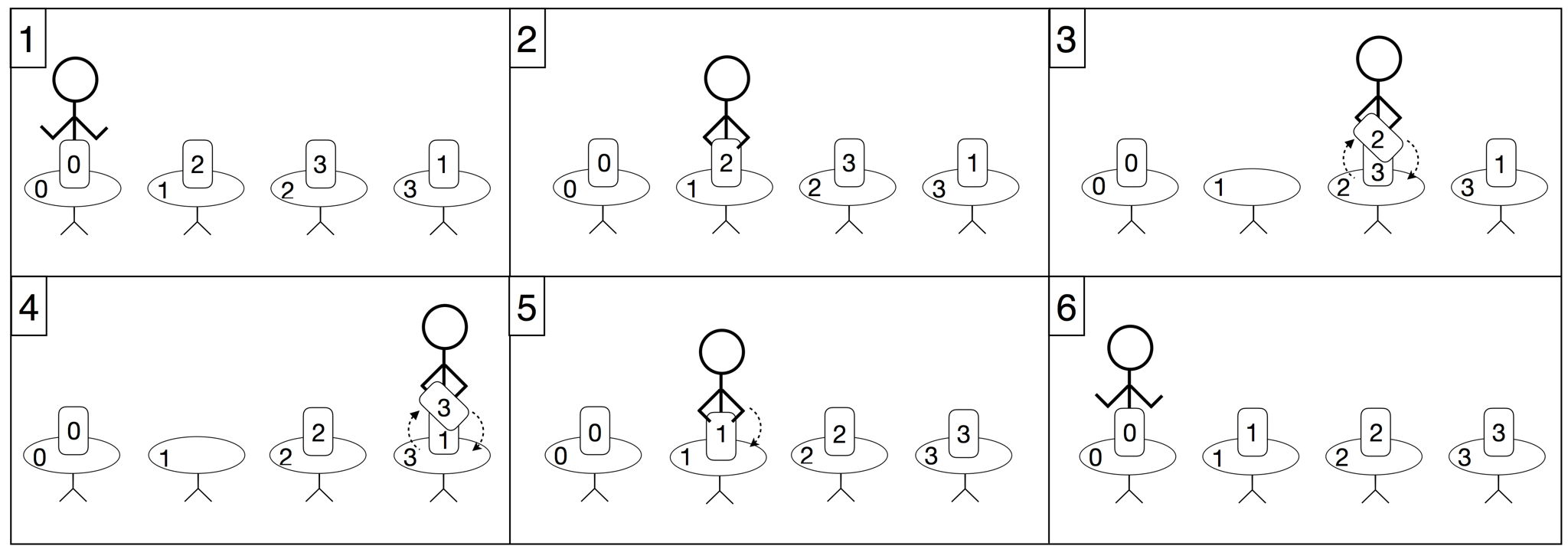시간 제한메모리 제한제출정답맞힌 사람정답 비율
2 초 1024 MB84191521.429%

## 문제

The city of Tehran is home to the National Library of Iran. The main treasure of this library is located in a long hall with a row of $n$ tables, labeled $0$ through $n-1$ from left to right. On each table there is one ancient handwritten book being displayed. These books are ordered based on their ages, which makes it hard for the visitors to search for books by title. So, the library manager has decided to sort the books in alphabetical order of their titles.

Aryan, a librarian, is going to do the job. He has created a list $p$ of length $n$, containing different integers from $0$ to $n-1$. This list describes the changes needed to rearrange the books into alphabetical order: for all $0 \leq i < n$, the book that is currently on table $i$ should be moved to table $p[i]$.

Aryan starts sorting the books at table $s$. He wants to return to the same table after finishing the job. Since the books are very valuable, he cannot carry more than one book at any time. While sorting the books Aryan will perform a sequence of actions. Each of those actions has to be one of the following:

• If he is not carrying a book and there is a book on the table he is at, he can pick up this book.
• If he is carrying a book and there is another book on the table he is at, he can switch the book he is carrying with the one on the table.
• If he is carrying a book and he is at an empty table, he can put the book he is carrying on the table.
• He can walk to any table. He may carry a single book while he does so.

For all $0 \leq i, j \leq n - 1$, the distance between tables $i$ and $j$ is precisely $|j-i|$ meters. Your task is to compute the minimum total distance Aryan needs to walk in order to sort all the books.

## 구현

You should implement the following procedure:

int64 minimum_walk(int[] p, int s)

• $p$ is an array of length $n$. The book that is on table $i$ at the beginning should be taken by Aryan to table $p[i]$ (for all $0 \leq i < n$).
• $s$ is the label of the table where Aryan is at the beginning, and where he should be after sorting the books.
• This procedure should return the minimum total distance (in meters) Aryan has to walk in order to sort the books.

## 예시

minimum_walk([0, 2, 3, 1], 0)In this example, $n=4$ and Aryan is at table $0$ at the beginning. He sorts the books as follows:

• He walks to table $1$ and picks up the book lying on it. This book should be put on table $2$.
• Then, he walks to table $2$ and switches the book he is carrying with the book on the table. The new book he is carrying should be put on table $3$.
• Then, he walks to table $3$ and switches the book he is carrying with the book on the table. The new book he is carrying should be put on table $1$.
• Then, he walks to table $1$ and puts the book he is carrying on the table.
• Finally, he walks back to table $0$.

Note that book on table $0$ is already in the correct place, table $0$, so Aryan does not have to pick it up. The total distance he walks in this solution is $6$ meters. This is the optimal solution; hence, the procedure should return $6$.

## 제한

• $1 \le n \le 1\,000\,000$
• $0 \le s \le n-1$
• Array $p$ contains $n$ distinct integers between $0$ and $n-1$, inclusive.

## 서브태스크

번호배점제한
112

$n \le 4$ and $s = 0$

210

$n \le 1000$ and $s = 0$

328

$s = 0$

420

$n \le 1000$

530

## 샘플 그레이더

• line $1$: $\;\; n \;\; s$
• line $2$: $\;\; p \;\; p \; \ldots \; p[n-1]$

The sample grader prints a single line containing the return value of minimum_walk.

## 출처• 문제를 만든 사람: Daniel Graf

## 제출할 수 있는 언어

C++17, C++14, C++20, C++14 (Clang), C++17 (Clang), C++20 (Clang)

## 채점 및 기타 정보

• 예제는 채점하지 않는다.